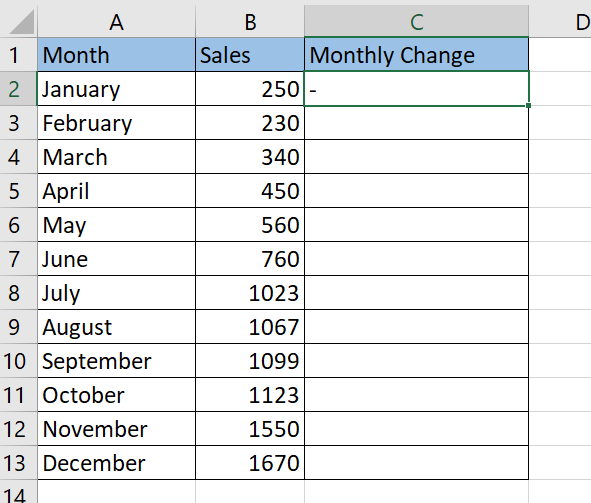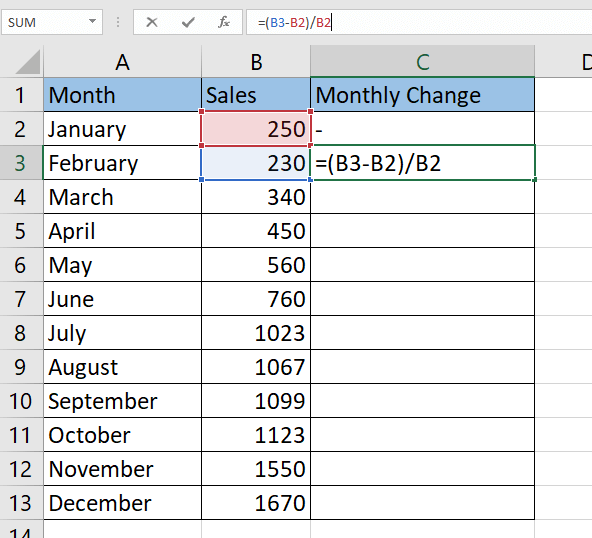# How to Calculate Percent Change in Excel

In this guide, we will explain how you can use a simple formula to calculate percent change in Excel.

Percent change is the change in value divided by the absolute value of the original value, multiplied by 100. We can use this calculation to understand how much an original value increased or decreased.

Percentage change is often used when comparing data over time and when analyzing differences in rates. Let’s take a look at a few quick examples where computing for percentage change is beneficial.

We can use percentage change to track the performance of stocks. Given a certain timeframe like 24 hours or seven days, you can use the percentage change formula to determine whether a stock has increased or decreased in value.

Discount rates are a type of percentage change. If you know the original and current product price or service, you can compute which product or service is most heavily discounted.

Let’s say we know that an apartment was valued at \$1,500,000 six months ago. If the current valuation today is \$1,900,000, what is the relative change of the house’s valuation?

We can compute for the percentage change by using the following formula:

`= (<new_value> - <old_value>) / <old_value>`

Plugging in the house prices, we get =(1,900,000 – 1,500,000) / 1,900,000 which returns 0.666 or a 66.6% increase in price.

Now that we know when to use the percent change formula, let’s look into how we can apply it to different situations by looking at an actual spreadsheet.

## Real Examples of Calculating Percent Change in Excel

Let’s take a look at a few examples of the percent change formula being used in an Excel spreadsheet.

The example below contains a table with monthly sales data. Column C uses a percent change formula to show how much the amount of sales increases or decreases each month. For example, February saw an 8% decrease in sales from January. The percentage format allows us to quickly see which months saw significant spikes in sales, such as March and November.To get the values in Column C3, we just need to use the following formula:

`=(B3-B2)/B2`

The second example below shows a list of video game titles and their original and discount prices. We used the percent change formula to determine which games are the most heavily discounted.The table reveals that title A is the most heavily-discounted item while title D has the lowest discount.

If you’re ready to try out the percent change formula in Excel, read our next section and follow our step-by-step guide!

## How to Calculate Percent Change in Excel

This section will guide you through each step needed to start using the percent change formula in Microsoft Excel. You’ll learn how we can use simple arithmetic to find out the relative change between two values.

In this example, we’ll be replicating the table containing monthly sales data. We’ll use the percent change formula to compute the relative monthly change in sales.

Follow these steps to start using the percent change formula:

1. First, we should leave the first cell in Column C blank. We have no prior monthly data to compare January to.2. We can now fill out the February monthly change cell with our percent change formula. In this example, cell B3 is our current value, and cell B2 is our old value.3. This gives us a decimal value that may either be positive or negative. We can convert this into a percentage by changing the format, as seen below.4. Your value in Column C should now be in the percentage format.5. We can fill the rest of the column by dragging down the formula in cell C3.In what situations should I use percentage change over absolute change?

While there are many situations where percent change feels intuitive, it can sometimes lead to misleading data.

For example, let’s say that a student increased his grade from 10 to 15 while another student raised it from 80 to 95. In absolute terms, the second student had a higher jump in score. Using percent change, the first student increased their score by 50%, and the second student increased by about 16%

Relative changes in larger numbers may appear less significant. Even when the absolute change is significant the relative change may look insignificant if it is a change on a larger number. When reporting on data, it’s best to present both the relative and absolute change to your audience.

That’s all you need to remember to calculate percent change in Excel. This step-by-step guide shows how easy it is to find the relative change of two values.

The relative percentage change formula is just one example of a mathematical formula you can apply in Excel. With so many other Excel functions out there, you can surely find one that suits your use case.

Are you interested in learning more about what Excel can do? Make sure to subscribe to our newsletter to be the first to know about the latest guides and tutorials from us.### Get emails from us about Excel.

Our goal this year is to create lots of rich, bite-sized tutorials for Excel users like you. If you liked this one, you'd love what we are working on! Readers receive ✨ early access ✨ to new content.

##### You May Also Like## How to Calculate WACC in Excel

This guide will explain how to calculate WACC in Excel using basic arithmetic. In other words, we need…## How to Add Calculated Field to Pivot Table in Excel

This guide will explain how you can add a calculated field to a Pivot Table in Excel. When…## How to Use VLOOKUP Function with Exact Match in Excel

This guide will explain how to use the VLOOKUP function for exact matches in Excel. VLOOKUP is a…## How to Use Linear Interpolation in Excel

This guide will discuss how to use linear interpolation in Excel.  Excel is an excellent tool to utilize…